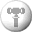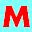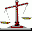# Counting The Math

 Sort by:Date Added Product Name Total Downloads Only Freeware and Open SourceMultiplication Master 1.1 Free multiplication tables practice software for all ages.Numbers Up! Volcanic Panic Windows 1.2.2 A fun math game for ages 4-15 covering basics of number facts and operations.2+2 2.1a A program aimed to help children's education in the area of basic math.Math Rescue 2.0 Mathematical game to improve your skills in addition, subtraction, multiplication and division.Math Homework Maker 1.0 A free software which can solve all your homework.Numbers Up! Volcanic Panic OSX 1.2.2 A fun math game for ages 4-15 covering basics of number facts and operations.Multiplication Facts 1.0 Practice times tables with digits 0 to 12.Easy Math 2.0 Exercises for Multiplication, Division, Subtraction, Addition, Fractions, etc.Addition Flash Cards Let your kids learn addition the easy way.Balance Keeper 1.1 Helps children practice addition, subtraction, logical thinking, basic algebra.